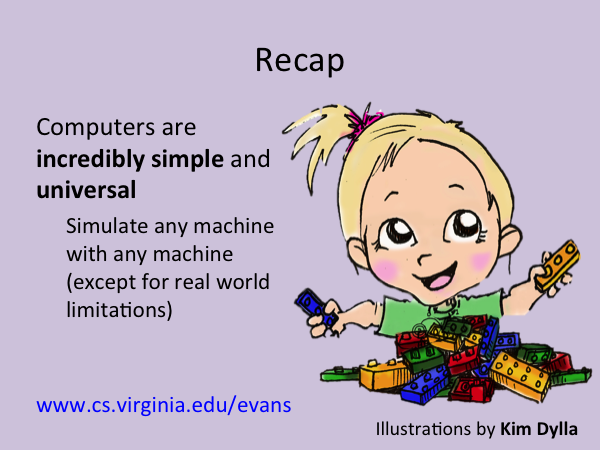# What Every Hacker Should Knowabout Theory of Computation

Hacker School (at eBay Office)
New York, New York
13 October 2014

Hacker School is a "retreat for programmers", where a remarkable group of curious and self-motivated people from a wide range of backgrounds put their lives on hold for 12 weeks to gather with like-minded people to learn about programming.

Before coming I gave them a list of possible topics to talk about, and I was pleasantly surprised when they selected theory of computation, both since of course, it is always great to be able to talk about theory (especially in the evening for one of the Hacker Schoolers 21st birthday), and because it gives me a not-as-shameless-as-usual opportunity to plug my favorite book. (I do, it seems, have a low barrier for shameless plugging, and find ways to plug it at most talks I give these days!)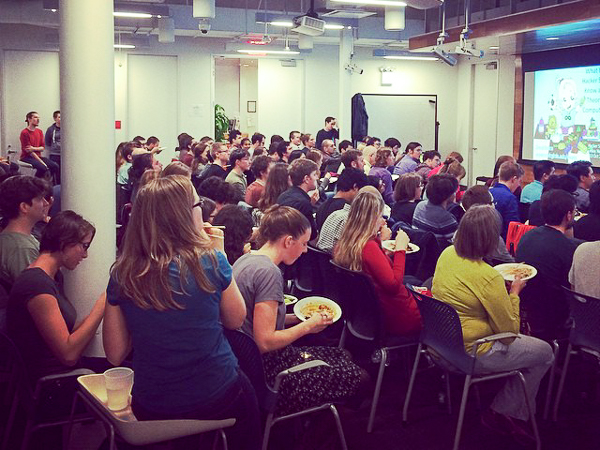If we want a theory about computers, we need to know what a computer is!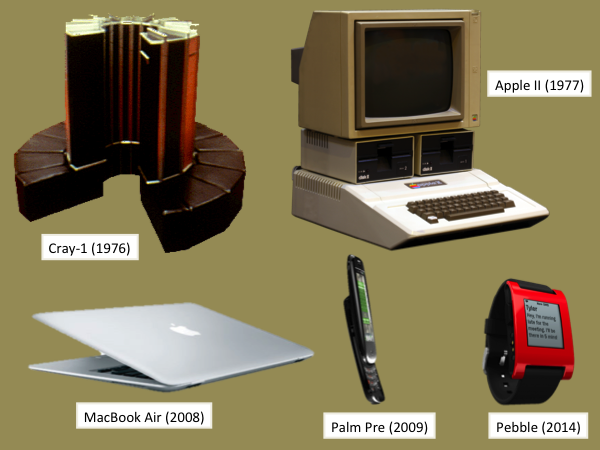Here are some of my favorites. Our theory better capture all of these (to some degree, although probably it shouldn't include the nice machine-heated bench provided by the Cray-1).Going further back, all of these seem like "computers" also. Colossus is (arguably) the first useful, electronic, programmable computer. It was constructed by the Allies at Bletchly Park to break Nazi codes during World War II. The Apollo Guidance Computer was (arguably) the first "personal" computer, built to be small enough to fit in an Apollo capsule for use by the astronauts. Trying to make a computer that was small enough to fit in a capsule and powerful enough to perform the calculations needed to get to the moon and back was the main impetus for the IC industry and what became silicon valley. The Honeywell Kitchen Computer had a somewhat less glorious history than the other two (but at least it did come with a two-week programming course!).Going back earlier, a "computer" was a person who is employed to compute, just like a "brick-layer" is a person who is employed to lay bricks, a "actor" is a person who is employed to act, and a "lawyer" is a person who is employed to lie.

Our model for a computer should be able to capture all of these things.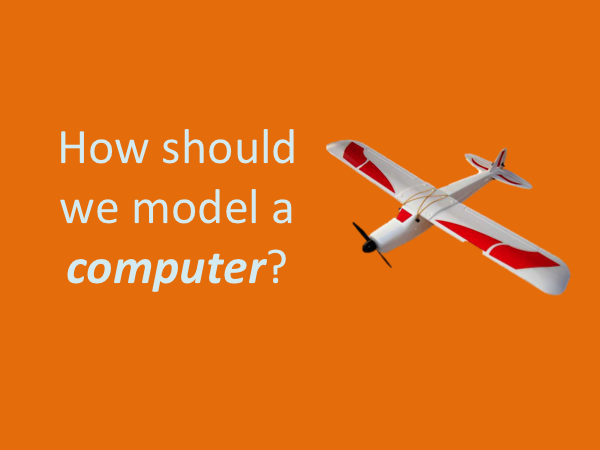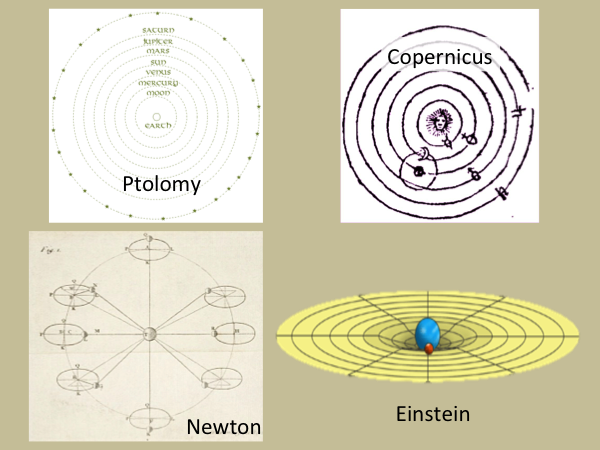Lots of people have attempted to construct models of the physical universe. None of them are correct, and some of them seem pretty silly to us today. But even Ptolomy's model was very useful (albeit misleading) in its day, and remains a useful model for developing planetarium projectors today.

A model is good if it approximates some aspects of reality well enough to make accurate predictions, while being simple enough for us to use it to reason with. The best model to use is not always the most accurate one, and depends on the task at hand. If you are building a bridge, Newton's model is probably the right one; for GPS satellites, you better use Einstein's model.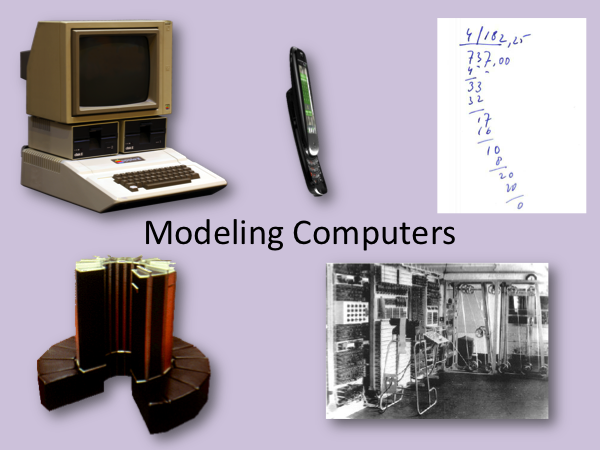We want a model of a computer that is able to approximate the behavior of machines ranging from a child computing with pencil and paper, to a modern smartphone, while being simple enough to reason with and understand.

It is a remarkable consequence of the universality of computation that a very simple model is able to usefully (but not accurately!) capture the computational capabilities of all of these.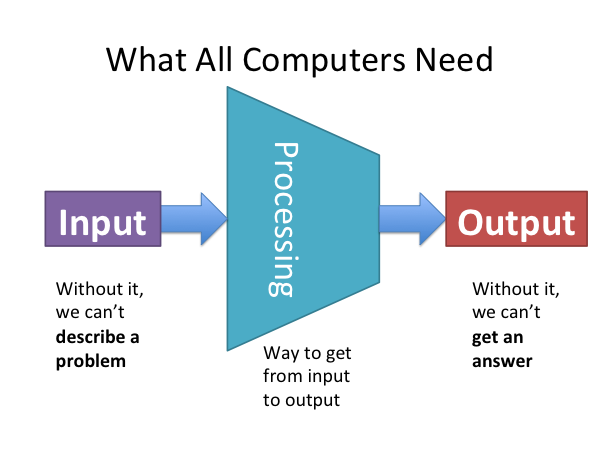All computers at least need three things: input (a way to feed a problem into the machine), output (a way to get results back from the machine), and processing (a way to get from the input to the output). Input and output seem easier to model than processing, so let's tackle these first.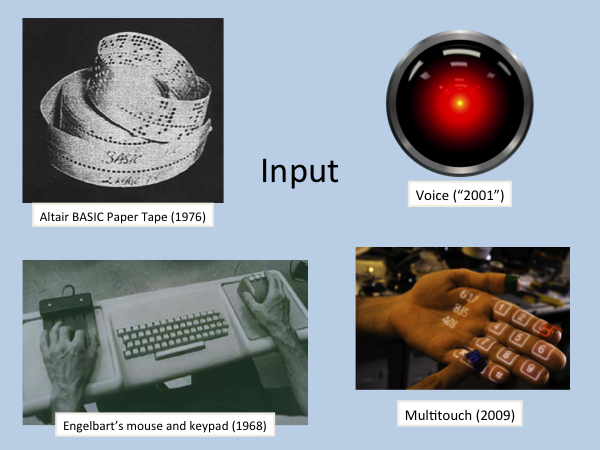There are lots of ways to get input into a computer, and the way a computer takes input has a big impact on our personal experience with it.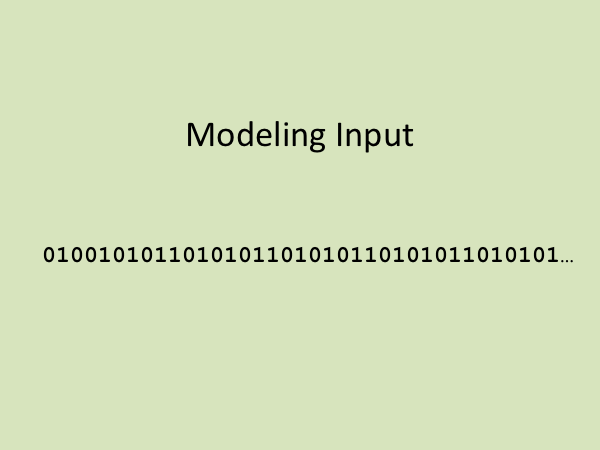But, we can model all of these with just a stream of bits.

(Its a bit harder to see how we can model interactive interfaces with just a string of bits, and we're missing a lot of what is important about those interfaces by doing this. But, we could imagine pre-recording all the input and writing it done as a string of bits. If the interaction is really important to model, we could break out computer into multiple steps, so for each step there is an input string, the computer does some processing and produces an output, and then there is a subsequent input → processing → output for the next user interaction.)Output is similar, and for many of the most interesting uses of computing today the output is not intended to be seen by a human, but instead to control some physical thing.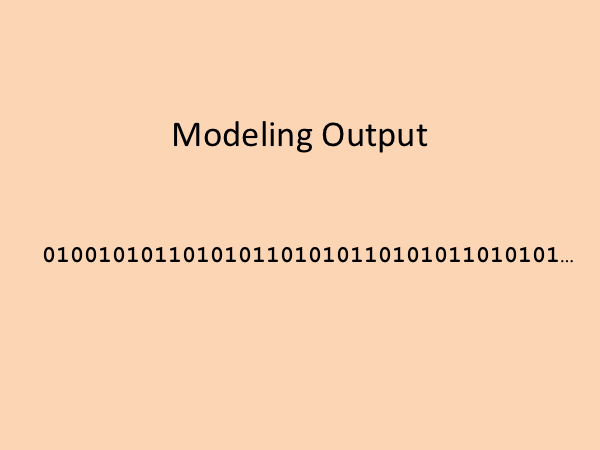But, we can still think of all of these as strings of bits.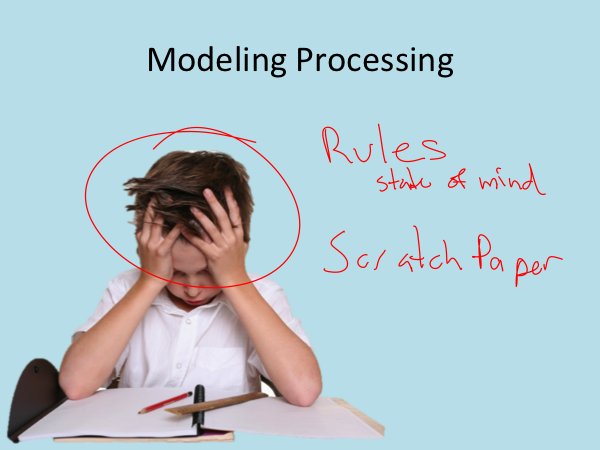Let's think about modeling the processing that our suffering 3rd-grader is doing solving long addition problems. The "computer" has some scratch paper for keeping track of what he is doing, with a pencil for writing symbols on the scratch paper.He can look at a small part of the scratch paper at a time. In his head, he (hopefully) knows the rules to follow for manipulating the symbols. He performs the addition in a sequence of small steps, each of which involves following a rule (e.g., look for the leftmost digits, add up two digits using a memorized lookup-table (e.g., (3, 5) → (8, 0); (3, 6) → (9, 0); (3, 7) → (0, 1); ...) keeping track of the result and carries on the scratch paper, move on to the digit to the right, etc.).This is very close to the model that Alan Turing came up with in the 1930s, which is still the most widely used and important model of a computer!

Indeed, Turing developed the model by modeling what a human computer does, and his purpose in developing the model was to understand the set of numbers that can be "computed". (We'll get to the specifics of Turing's model soon, and why some numbers cannot be computed.)

Turing's full paper is here: On Computable Numbers, with an Application to the Entscheidungsproblem, Proceedings of the London Mathematical Society, received 28 May 1936 (appeared in 1937). Despite the rather intimidating title (Entscheidungsproblem just means "decision problem" in German) and some difficult notation choices, the paper is actually very readable.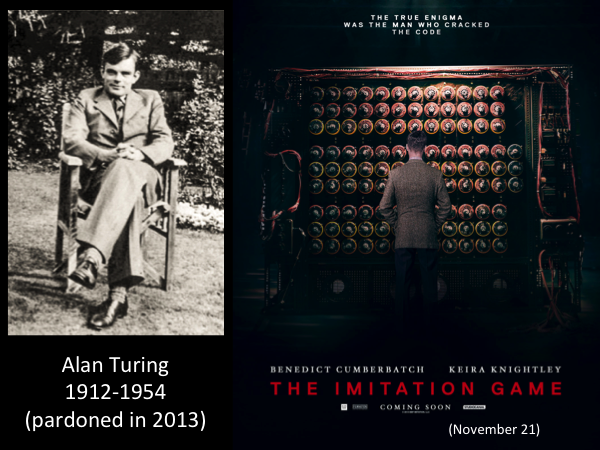I was pleased that nearly the entire audience was familiar with Turing and his role in World War II and subsequent persecution, but I think this is an atypical audience of Hacker Schoolers. Most of the US population remains woefully ignorant of this history, including many CS students I encounter.

I hope and expect that to change soon, if the upcoming movie, The Imitation Game, is a good as the early reviews indicate. The film is scheduled for release in the US on 21 November 2014. (I haven't seen it, but don't expect it will include a lot of details on Turing's model of computation or proof about uncomputable numbers.)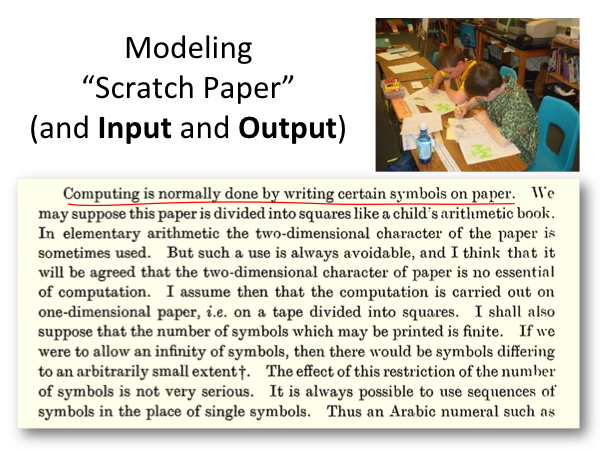To model the "computer", we need to model the scratch paper, and how the in-head processing works.

Here, Turing argues that we although we could draw infinitely many different squiggles on the paper not confined to squares or a limited set of symbols, there are only a finite number of scribbles that could be reliably distinguished. Hence, we can limit our model to a finite set of symbols that can be written in defined squares on the scratch paper.We can also turn the two-dimensional paper into to a one-dimensional tape. One easy way to see this is just slide the paper into one-square cuts, and stick them all together, making a one-dimensional tape.

Actual scratch paper has limited size (as does actual memory in a modern computer), but for our model, we don't need any such artificial limits. Instead, the tape is infinite, extending forever in both directions.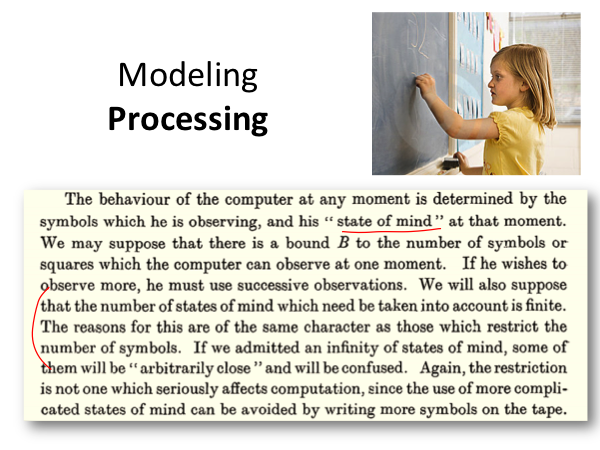The other thing we need to model is the computer's state of mind. Turing argues that there can only be a finite number of different states of mind, since if there were too many they would be too close to each other and the "computer" (remember this is a human doing computations) would not be able to avoid getting them confused.The computation can be divided into a sequence of distinct steps ("simple operations"), each of which makes a small change to the scratch paper and changes the state of mind. The model assumes a "tape head" which is the focus on the scratch paper, and is limited to a single square.

In one step, the machine can:

1. Read the symbol that is in the current square.
2. Follow a rule that depends on the symbol read and the current state of mind. The rule describes three changes that are done as a results of this step:
1. Write a symbol into the current square (replacing whatever was there at the beginning of the step),
2. Move the tape head one position to the left or right,
3. Change the state of mind to any other state.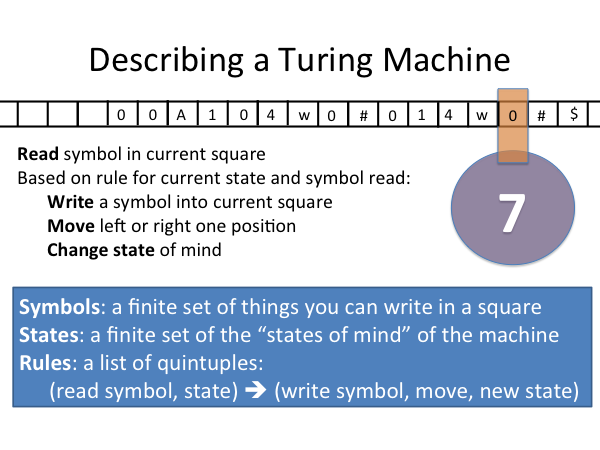Note that this means that we can write down the complete description of a Turing machine just by listing the rules, which are just quintuples.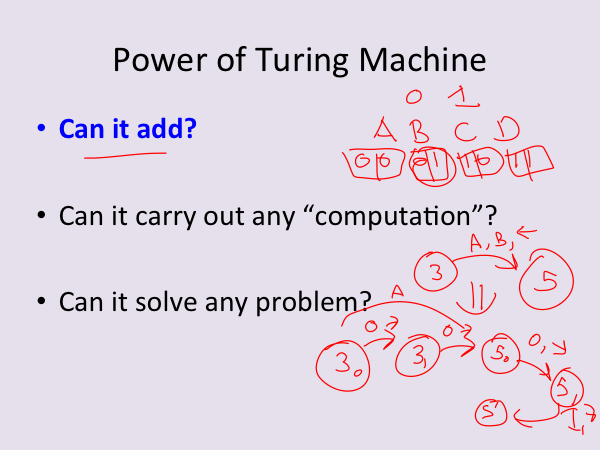To get a sense of the power of our Turing machine model, let's look at some computations and see if it can do them. Intuitively, this should seem like such a simple model it is surprising it can do anything --- it has no notion of numbers, loops, arithmetic, comparison, etc., just a way to lookup rules in a table and keep track of state (both in the scratch tape and state-of-mind).

The scribbles on the slide are in response to a question about whether it matters to limit the machine to only two symbols, as we had done in the initial modeling of input and ouptut as binary strings. Having more than two symbols does not provide any additional computing power, since we can always encode any number of different symbols using binary, just needing N bits to encode up to 2N different symbols.

So, if our symbols set is the four letters, {A, B, C, D}, we can encode them as the two-bit sequences, {00, 01, 10, 11}. Then, we would need to change the machine rules to be able to read in two symbols and keep track of them to transition into one of four states corresponding to reading each of the four letters. Thus, we'll need a lot more states to construct the machine than we would if we could have more symbols, but it is always possible to convert a machine using a large input alphabet into one that uses only two symbols.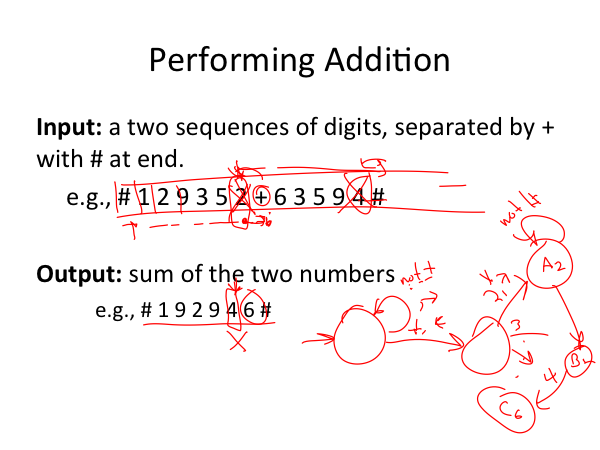As an example to get a sense of the power of Turing's model, let's see if we can design a machine that can add any two decimal numbers.

First, we need to formalize the problem by describing the input format and desired output.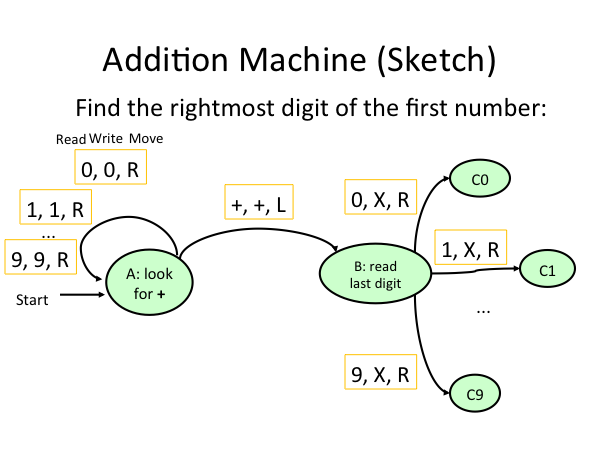Here's a rough sketch for our adding machine. The strategy is to first look for the + symbol to find the end of the first number, by moving right on all other symbols. Then, move left one square to get to the last digit. Read that digit and cross it out (this will be important so we can continue to add the remaining digits), moving right and entering a different state for each digit read (this is necessary to keep track of which digit we read).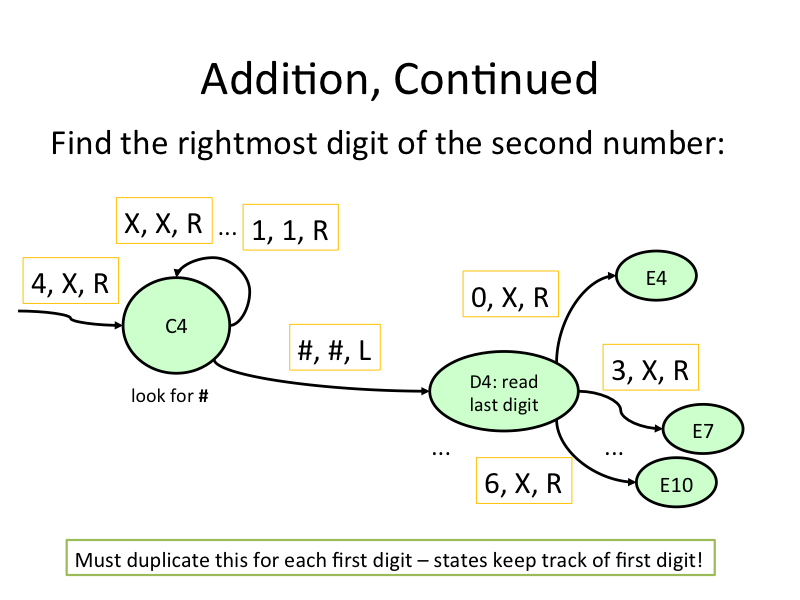Now, move right across the tape looking for the # that marks the end of the second number (state C4 here; we could need similar states for each first number read). Then, move left one square to read the last digit of the second number (entering state D4).

Then, based on the number read, move to a state that keeps track of the resulting sum, and cross out that digit (e.g., state E7 since we are adding 4 and 3 in the ones places). From here, we would need to move to a new place on the tape where the output sum will be written and write the 7. Then, return to the leftmost edge of the tape, and repeat the process for the next digit.

A complete machine would need to also keep track of carries, and handle the case where one number runs out of digits before the other one does. But, hopefully this is enough to be convinced that building an adding machine that can add any two numbers is possible. (Enthusiastic readers are encouraged to work out all the details, and try running your machine in a Turing machine simulator.)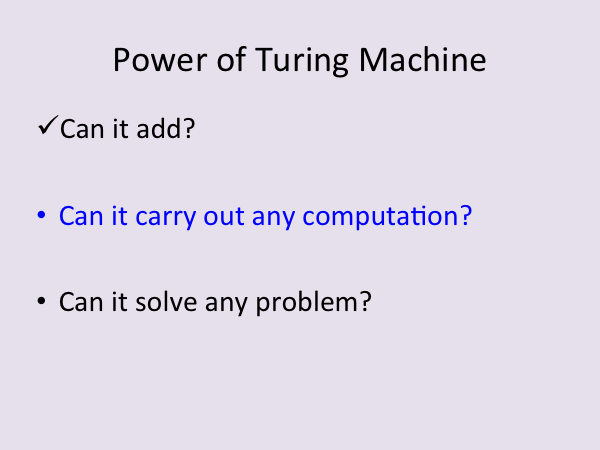Being able to add is a pretty good start to becoming convinced that a Turing machine can perform many computations. It is fairly clear to see that if you have a machine that can add, you should be able to also construct one that can multiply (by doing repeated additions, at worst), and then one that can divide, as well as machines that can do comparisons, and do string and set operations.

We certainly don't want to have to actually design all these machines, though. Instead, we consider the possibility of building one Turing machine that can simulate ever other Turing machine.

The question, "Can it carry out any computation?" doesn't really make sense since we don't have a good definition of "any computation", only our intuition about what representative computers can actually do. Without a formal definition of "any computation", we end up with circular definitions like "computation is what a Turing machine can do", so "a Turing machine can do any computation". Nevertheless, looking at simulating other abstract machines will build our confidence that this model captures our intuition of what a computer can do.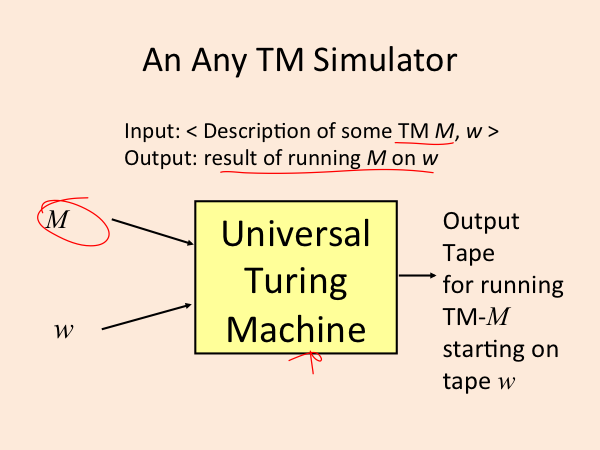A universal machine is one that can simulate every other machine. So, to design a Universal Turing Machine, we need to design a machine that takes a description of any Turing Machine as its input, along with a description of an input, and simulates the machine described, producing the output that would be produced by that machine.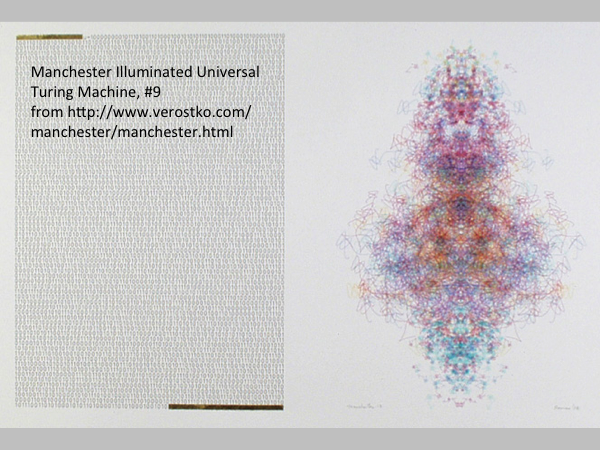Turing's paper describes one way to construct such a machine, going into detail on how you represent the input machine and the rules needed to simulate. There are lots of other ways to construct universal machines, some even look artistic.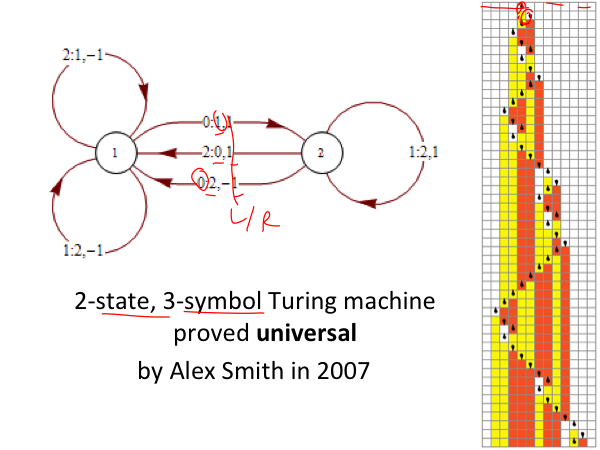This 2-state, 3-symbol machine is proven to be universal. (Getting such a small machine to work requires a lot of complexity in how the simulated machine and input are encoded, so raises some controversy over whether the encoding is actually doing the computation, not the simple machine here, but there is fairly strong agreement that the machine is universal.)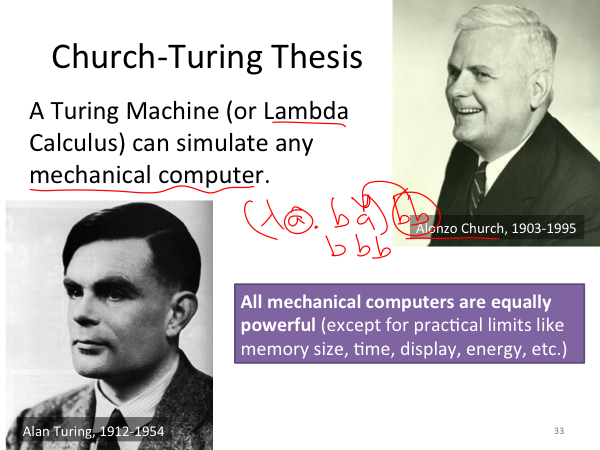The Church-Turing Thesis is that all standard computers are equally powerful. This isn't the kind of statement that can be proven, since it depends on an infomal notaion of what a "standard computer" is.

In the 1930s, there were two models of computing that emerged around the same time: Alan Turing's model that we have been discussing, and Alonzo Church's lambda calculus model. The models are very different, but equivalent: each model can perfectly simulate the other one.

Lambda calculus models computation using simple rules for string substitution. The main rule is beta reduction (shown in the scribble on the slide), which is sort of like evaluating a function call. This lecture explains lambda calculus, and sketches what you would need to do to show it is equivalent to Turing's model.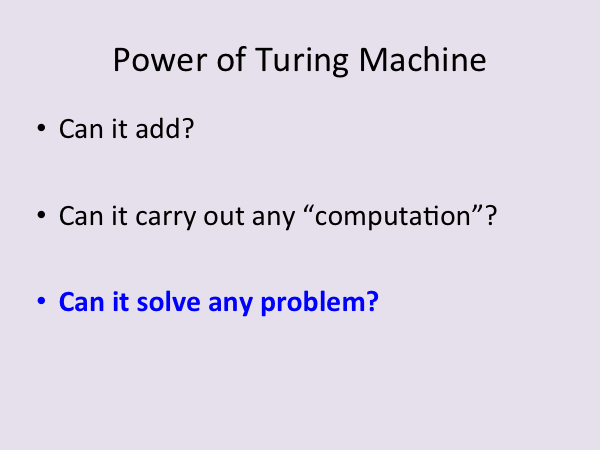At this point, you should start to be convinced that this simple computing model is actually amazingly powerful! It seems like it can actually compute anything.

Next, we'll see that there are problems that cannot be solved by any Turing machine (and, following the Church-Turing thesis, by any standard computer).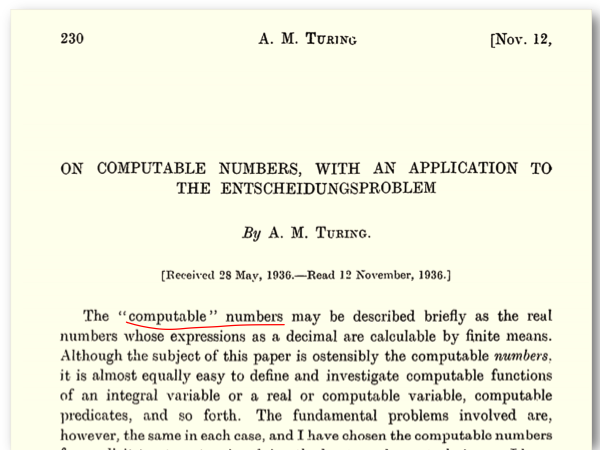Getting back to Turing's paper, his goal in developing the model of computation is to answer the question what numbers can be computed.

A number can be computed if an arbitrarily precise estimate of that number can be output by a computer (Turing machine). Let's assume a decimal representation. So, a number like 2 is computable since it is trivial to construct a one-state Turing machine that outputs 2. The number 1/3 is also computable, even though its decimal representation cannot be written down complete. We can construct a machine that outputs 0.3333333... and keeps writing more 3s, producing a number that is arbitrarily close to 1/3.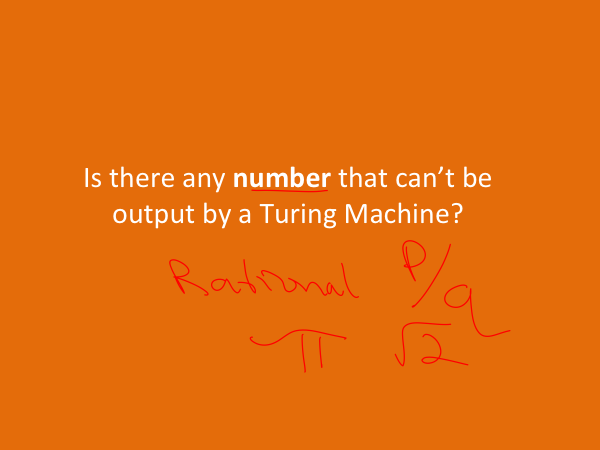What about all rational numbers? A number is rational if it can be written as p/q where p and q are integers. Since we can design a Turing machine that does division, we can compute the decimal representation of any rational number.

Irrational numbers are numbers that have no rational representation like π and the square root of 2. Some irrational numbers, including π and √2 can be computed by a Turing machine. For example, Leibniz found the forumal for computing π as:Since we can make a Turing machine that does addition, subtraction, and division, we can use this formula to generate as many digits of π as we want, computing the irrational value with unlimited precision.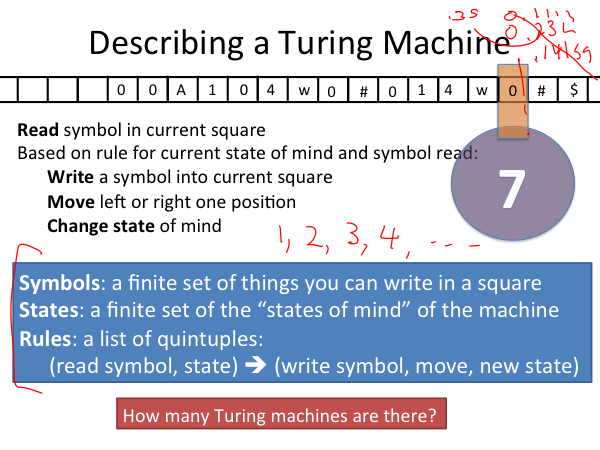But there must be some real numbers that cannot be computed by any Turing machine.

The reason for this is that the number of real numbers exceeds (by a huge amount!) the number of Turing machines. Both numbers are infinite, so if you haven't seen arguments involving different types of infinity be prepared to have your mind blown.

The number of Turing machines is the same as the number of whole numbers: we can write down all the Turing machines in order using some grammar rules about how to produce all possible Turing machines, and make a one-to-one mapping between the whole numbers and the Turing machines.

Georg Cantor showed there is no one-to-one mapping between the real numbers and the whole numbers, so there are more real numbers than there are whole numbers. An informal version of his diagonalization argument starts by assuming that there is such a mapping, and showing how to create a real number that is not included. If there is a one-to-one mapping between the real numbers and whole numbers, we could find some order of writing down all the real numbers like this (for simplicity, assume we're only worried about numbers between 0 and 1, and each is preceeded by 0.):

1.   2500000000000...
2.   3333333333333...
3.   1415926535897...
4.   28318530717958...
5.    ...

We can create a real number that is not in the table by going down the diagonal, setting the corresponding digit of the new number to be different from whatever is there. For example, 0.3422... must not be in the original table since it differs from each number in that table at at least one position.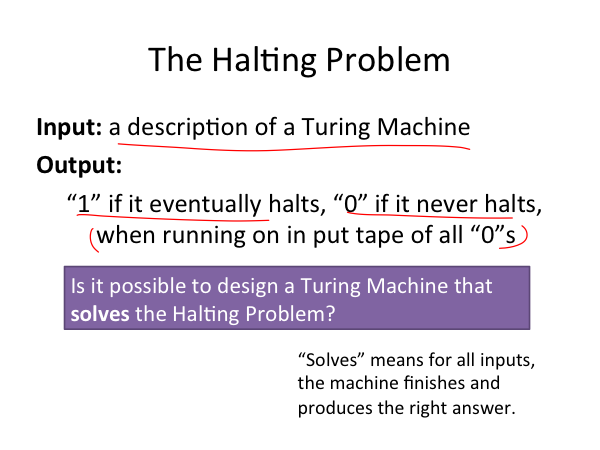This contradicts the claim that there exists a one-to-one mapping between the real numbers and integers, showing that there are some real numbers that cannot be computed by any Turing machine. But, it doesn't tell us any particular number that cannot be computed by a Turing machine, just that such numbers must exist.

The most famous example of a problem that cannot be solved by any Turing machine is known as the Halting Problem. Turing's paper introduced this problem (in a slightly different version that it is presented here) and proved that it was not computable.

The problem is simply stated: take as input a description of a Turing machine, and produce as output 1 if the input machine would eventually halt and 0 if the input machine would never halt (we'll assume the initial input tape is full of 0s, but this isn't important for the problem, just something we need to specify).

A machine that solves this problem would be able to always finish, and always produce the correct output result. We'll prove that no such machine can exist by showing that its existence would lead to a very strange paradox.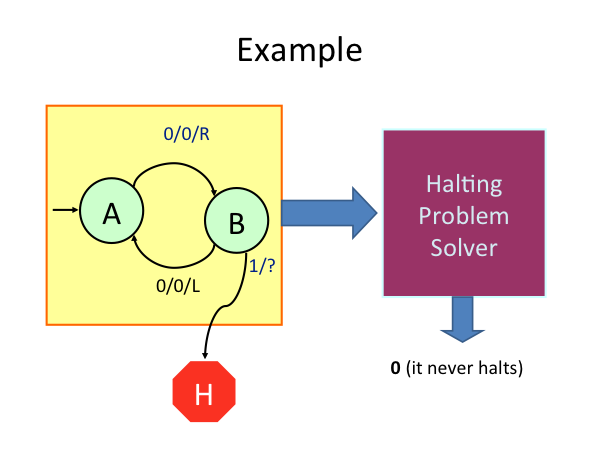A machine halts if it reaches a state where there is no rule for continuing. For example, with the machine shown if there is no rule for state B and tape symbol 1, the machine would halt if it ever reads a 1 on the tape while in state B.

We can certainly solve the Halting Problem for some inputs. For example, it is easy to look at the machine shown as the input machine here and conclude that it would run forever and the Halting Problem Solver machine should output 0.

Note that we can't do this by just simulating the input machine, though (even though we know it is possible to simulate any Turing machine, since that's what a Universal Turing Machine does). The reason for this is we don't know when to stop simulating and decide the simulated machine never halts. We could try running for, say, 3 quadrillion steps, and conclude that the simulated machine will never halt if it is still running after that. But, I can design a machine that runs for 3 quadrillion steps and then halts on the next step, so such an approach would not always produce the correct answer.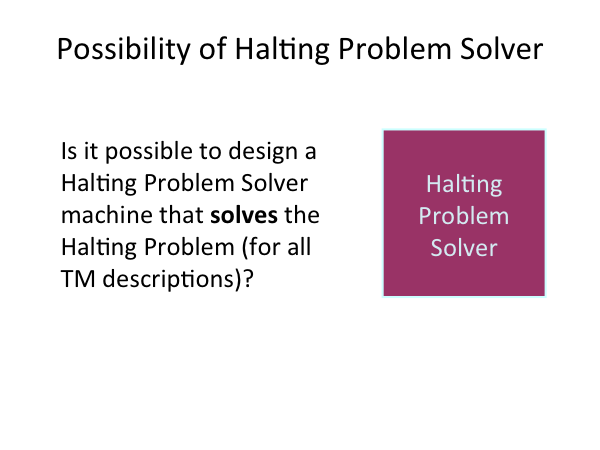But knowing that the straightforward simulation approach does not solve the problem is not enough to know that there is no way to solve it. Maybe there is some way to analyze the rules of the machine to detect if has any cycles (a machine with no cycles in the states is guaranteed to halt, but just because a machine has cycles does not mean it will never halt - recall the addition machine earlier, which has lots of cycles, but will always eventually halt).

So, to prove that there is no Halting Problem Solver machine, we need to show that having one would lead to a bizarre contradiction.

The trick is to construct a machine that uses the Halting Problem Solver machine as the input. Since we can describe any Turing machine as the input, and we are starting by assuming the Halting Problem Solver exists, using a description of the Halting Problem Solver as the input machine is perfectly reasonable.The Halting Problem Solver machine must halt on all inputs (if it is a value solution to the Halting Problem, it is required to always halt). This means there must be one of more states in the Halting Problem Solver machine that halt. We can simplify things by adding rules for all missing states and inputs that all transition to one new state we'll call F (for final).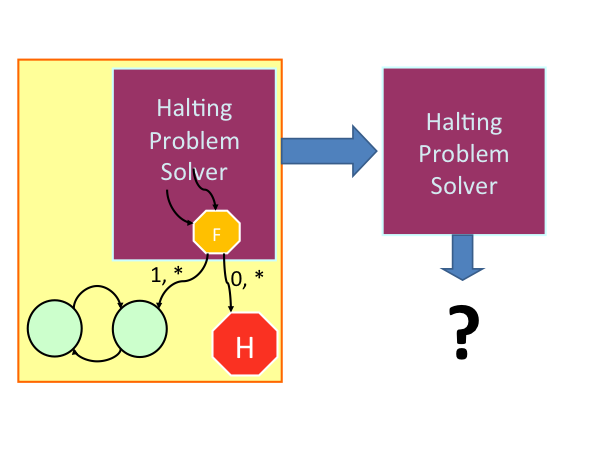Now, lets add some new transition rules for F that depend on the value written on the tape (which is the output of the Halting Problem Solver machine, with value 1 if the input machine would halt and value 0 otherwise).

For symbol 1, we'll add transitions to a loop that never halts; for symbol 0, we'll just transition to a halting state.

What should the output of the Halting Problem Solver be for the new machine?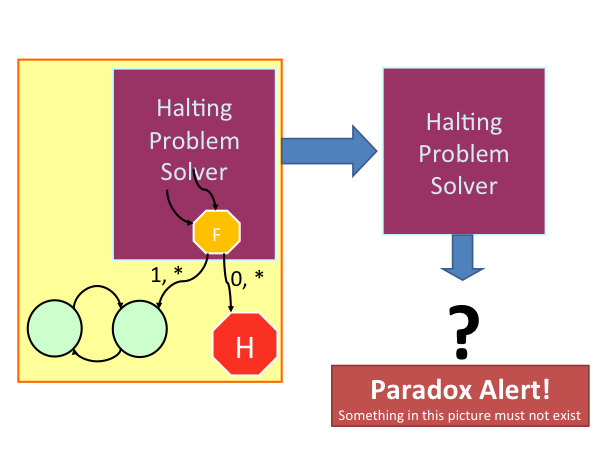There's no sensible output!

If it outputs 0, that means the input machine never halts, but that would mean it transitions from F to the halting state and halts! But, if it outputs 1, that means the input machine does halt, but that means it transitions from F to the looping states, and doesn't halt!

We have a paradox, so something here must not exist. Since this is an informal argument, its enough to say that it seems like the only thing that couldn't exist here is the Halting Problem Solver since everything else in our computing model is so simple. Thus, we know there is not Turing machine that can solve the Halting Problem.We don't need Turing machines to see the paradox — here's the same argument using Python code. The difficulty with this version is that maybe it isn't the halts function that cannot exist because of the paradox. Perhaps while loops don't really exist, or function calls in Python. They seem to exist because we can use them in programs that work, but that doesn't mean they strongly exist (that is, they might just work some of the time, but be broken in complex ways that fail here).Turing's model is useful for reasoning about computation abstractly, and understanding some of its fundamental limits. But, don't forget that its just a model.

Real computers are quite different. They are physical machines that operate in a physical word. Computation requires energy and generates heat. Most modern processors can also do some things that cannot be done by Turing machines, most notably produce randomness. Everything a Turing machine does is completely determined by its rules and input. Most modern processors have physical sources of randomness that can be used to generate cryptographic keys (that better be unpredictable to be secure).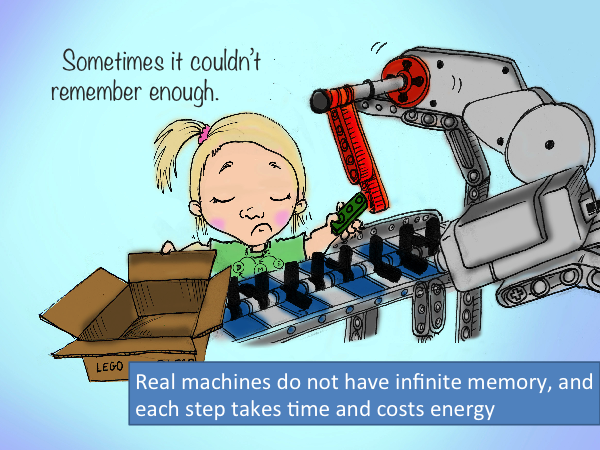All real machines also have finite memory, and take some time to execute each steps (hence, have a limited number of steps they can finish before the heat death of the universe, or more importantly, before the programmer expires). This means problems that are computable by abstract Turing machines, may not be practical to compute on real computers.